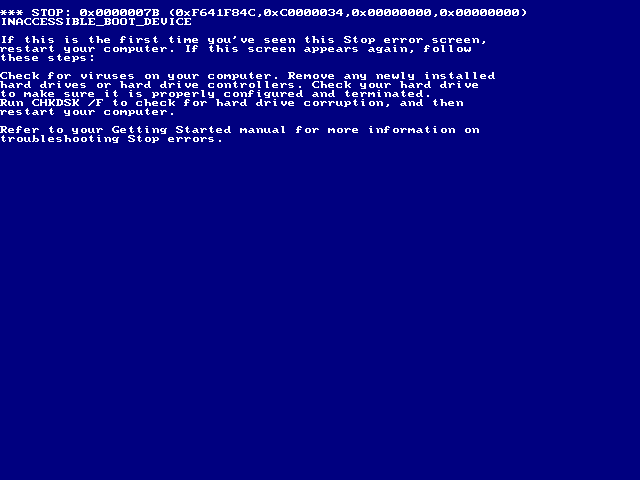# Just Make Sure Your Computer Doesn't Crash$10000$ players are participating in a round-robin tournament (each player plays against another player exactly once). Each player in the tournament is assigned a popularity index, a positive integer between $1$ and $100$ inclusive. The number of tickets sold in the game between two players is equal to the product of their popularity indices. Find the last three digits of the total number of tickets sold in the tournament.

Example
Say players $A,B,C$ are participating in a tournament. The following table lists their popularity indices. $\begin{array}{|c|c|} \text{Player} & \text{Popularity index} \\ \hline A & 5 \\ \hline B & 7 \\ \hline C & 8 \end{array}$ The following table lists the number of tickets sold in each game. $\begin{array}{|c|c|} \text{Game} & \text{Number of tickets sold} \\ \hline \text{A vs B} & 5 \times 7 = 35 \\ \hline \text{B vs C} & 7 \times 8= 56 \\ \hline \text{C vs A} & 8 \times 5 = 40 \end{array}$ The total number of tickets sold is $56+40+35 = 131.$

Input file
This file contains $10000$ lines with a positive integer between $1$ and $100$ in each line. The number written in the $i^{\text{th}}$ line denotes the popularity index of player $i.$

×

Problem Loading...

Note Loading...

Set Loading...